11.5.2 Step 2: Delete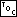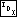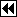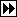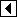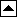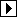```431. <Step 2: Delete RTAVL node 431> =
if (p->rtavl_link == NULL)   {
if (p->rtavl_rtag == RTAVL_CHILD)
{         <Case 1 in RTAVL deletion 432>       }
else       {         <Case 2 in RTAVL deletion 433>       }
} else   {
struct rtavl_node *r = p->rtavl_link;
if (r->rtavl_rtag == RTAVL_THREAD)
{         <Case 3 in RTAVL deletion 434>       }
else       {         <Case 4 in RTAVL deletion 435>       }
}

tree->rtavl_alloc->libavl_free (tree->rtavl_alloc, p);
```

This code is included in 429.

##### Case 1: p has a right child but no left child

If the node to be deleted, p, has a right child but not a left child, then we replace it by its right child.

```432. <Case 1 in RTAVL deletion 432> =
pa[k - 1]->rtavl_link[da[k - 1]] = p->rtavl_link;
```

This code is included in 431 and 470.

##### Case 2: p has a right thread and no left child

If we are deleting a leaf, then we replace it by a null pointer if it's a left child, or by a pointer to its own former right thread if it's a right child. Refer back to the commentary on <Case 2 in right-looking RTBST deletion 385> for further explanation.

```433. <Case 2 in RTAVL deletion 433> =
pa[k - 1]->rtavl_link[da[k - 1]] = p->rtavl_link[da[k - 1]];
if (da[k - 1] == 1)
pa[k - 1]->rtavl_rtag = RTAVL_THREAD;
```

This code is included in 431 and 471.

##### Case 3: p's left child has a right thread

If p has a left child r, and r has a right thread, then we replace p by r and transfer p's former right link to r. Node r also receives p's balance factor.

```434. <Case 3 in RTAVL deletion 434> =
r->rtavl_rtag = p->rtavl_rtag;
r->rtavl_balance = p->rtavl_balance;
pa[k - 1]->rtavl_link[da[k - 1]] = r;
da[k] = 0;
pa[k++] = r;
```

This code is included in 431.

##### Case 4: p's left child has a right child

The final case, where node p's left child r has a right child, is also the most complicated. We find p's predecessor s first:

```435. <Case 4 in RTAVL deletion 435> =
struct rtavl_node *s;
int j = k++;

for (;;)   {
da[k] = 1;
pa[k++] = r;
if (s->rtavl_rtag == RTAVL_THREAD)
break;

r = s;
}
```

This code is included in 431.

Then we move s into p's place, not forgetting to update links and tags as necessary:

```436. <Case 4 in RTAVL deletion 435> +=
da[j] = 0;
pa[j] = pa[j - 1]->rtavl_link[da[j - 1]] = s;

if (s->rtavl_link != NULL)
else   {
```437. <Case 4 in RTAVL deletion 435> +=